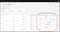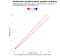# In Parallel

## Distributional assumptions are important for hypothesis testing.

Adobe Target is a popular website optimisation tool. The tool can serve different versions of a website or mobile app to different people. Analysts can then assess which version performs better on specified measures.

This article is about the statistical testing that Adobe Target uses. I also look at why distributional assumptions matter.

# A/B Testing

Digital analysts are often concerned with the ‘conversion’ from specified pages. For example, what proportion of users proceed from a page to a product application form?

User experience experts and analysts seek to improve digital experiences. This is to increase conversion and reduce conduct risks like mistaken sales.

According to its documentation, Adobe Target has run two tests:

• Difference: the difference of two independent proportions. The initial hypothesis is that the difference is zero and follows a t-distribution.
• Lift (ratio): the relative difference of two independent proportions minus 1. This time, the null hypothesis is the lift is zero and has a Normal distribution.

To illustrate, the first version of a page has a conversion rate of 1.0%. The second has a conversion rate of 1.3%. That is a difference of 0.3 points, and a lift (relative increase) of 30%.Adobe Target shows a confidence interval for the relative difference. (Image: Adobe)

# Distributional assumptions

In classical statistics, hypothesis testing seeks to establish differences through contradiction.

The p-value plays a crucial role. We start by assuming a specified model. Next, we calculate the probability of seeing a statistic as extreme or more as the observed value. This is the p-value.

A small p-value indicates incompatibility between the model and observed data. This incompatibility has many potential causes.

It could be an unusual event. Modelling assumptions might be incorrect. Errors in data collection procedures could have occurred. Researchers might have selected this particular value for presentation.

The targeted hypothesis is only one aspect of the model.

Misuse, misunderstanding and misinterpretation has led the American Statistician Association to state:

No single index should substitute for scientific reasoning.

Distributional assumptions matter. The specified model is part of how p-values are defined. Imagine the two different tests are run in parallel. Different approximations are being used. These parallel tests have parallel p-values with parallel conclusions.Colours diverging from white show the difference in calculated p-values. (Image: ggplot2)

I ran the calculations with 10,000 units in each sample. There are differences between these calculations close to equality. We can see two colour rays (showing difference) shooting from the origin.

There are larger differences when the two proportions are both similar and close to zero. This may be where the two approximations are not performing well. The ratio of two independent variables may not be well-behaved. Normal approximations to the ratio of two independent Normal variables can exist.

Sometimes, this difference is enough to mean only one of the two p-values is below 0.05. This is the widespread conventional threshold.

There is the pernicious practice of classifying test results as ‘significant’ and ‘non-significant’. The same data — with different models — gives different ‘conclusions’.

Researchers should be aware of the differences between statistical tests. These p-values being ‘small’ should not be the lone crux of organisational decisions. Estimating effects and their uncertainty helps interpret, communicate, and use digital analysis.

This blog looks at the use of statistics in Britain and beyond. It is written by RSS Statistical Ambassador and Chartered Statistician @anthonybmasters.

## More from Anthony B. Masters

This blog looks at the use of statistics in Britain and beyond. It is written by RSS Statistical Ambassador and Chartered Statistician @anthonybmasters.